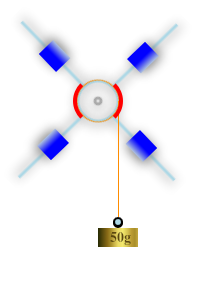# Oberbeck Pendulum

Oberbeck’s pendulum is an instrument for investigation rotational motion of rigid body. The instrument is widely used in laboratory of mechanics.  The pendulum consists of pulley, four crossed sticks,  and four cylinders on sticks. A thin flex wire is wrapped on the pulley. When you hang the load on the end of the wire, the pendulum begins to rotate. Angular acceleration of the Oberbeck’s pendulum depends on moment of inertia,  torque and mass of loads.The OBERBECK PENDULUM software is a simulator for Oberbeck’s pendulum. In order to execute experiment on OBERBECK PENDULUM, you have to hang loads on the bottom end of string.   Newton’s second law for rotation is:

$$I \frac{d \vec \omega}{dt} = \vec M$$

Here $\vec M$ is net external torque, $I$ is moment of inertia of the pendulum, $\omega$ is angular speed of the pendulum.

$$\vec M = \vec R \times \vec T$$

$$m \vec a = m \vec g + \vec T$$

$$ma = mg – T$$

$$T = mg – ma = m(g – a)$$

$$I \frac{d \omega}{d t} = Rm(g-a)$$